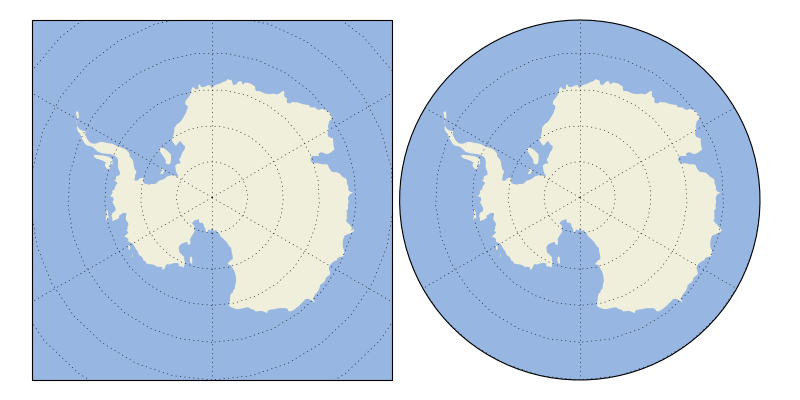Gallery

arrows example

always_circular_stereo example¶import matplotlib.path as mpath
import matplotlib.pyplot as plt
import numpy as np

import cartopy.crs as ccrs
import cartopy.feature

def main():
fig = plt.figure(figsize=[10, 5])
ax1 = plt.subplot(1, 2, 1, projection=ccrs.SouthPolarStereo())
ax2 = plt.subplot(1, 2, 2, projection=ccrs.SouthPolarStereo(),
sharex=ax1, sharey=ax1)
left=0.04, right=0.95, wspace=0.02)

# Limit the map to -60 degrees latitude and below.
ax1.set_extent([-180, 180, -90, -60], ccrs.PlateCarree())

ax1.gridlines()
ax2.gridlines()

# Compute a circle in axes coordinates, which we can use as a boundary
# for the map. We can pan/zoom as much as we like - the boundary will be
# permanently circular.
theta = np.linspace(0, 2*np.pi, 100)
center, radius = [0.5, 0.5], 0.5
verts = np.vstack([np.sin(theta), np.cos(theta)]).T
circle = mpath.Path(verts * radius + center)

ax2.set_boundary(circle, transform=ax2.transAxes)

plt.show()

if __name__ == '__main__':
main()